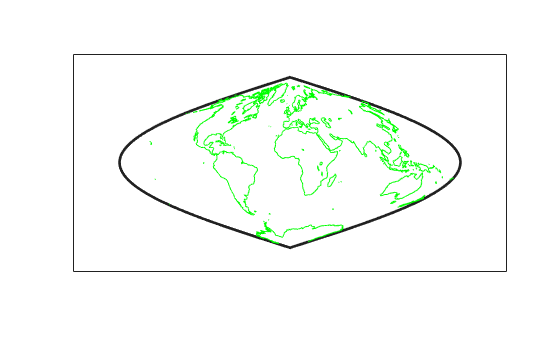# plotm

Project 2-D lines and points on map axes

## Syntax

``plotm(lat,lon)``
``plotm([lat lon])``
``plotm(lat,lon,LineSpec)``
``plotm(lat,lon,Name,Value)``
``h = plotm(___)``

## Description

`plotm` is the mapping equivalent of the MATLAB® `plot` function.

````plotm(lat,lon)` displays projected line objects on the current map axes. `lat` and `lon` are the latitude and longitude coordinates, respectively, of the line object to be projected. This ordering of latitude then longitude is standard geographic usage. However, this ordering is conceptually reversed from the MATLAB `line` function, in which the horizontal (x) coordinate comes first.```
````plotm([lat lon])` allows the latitude and longitude coordinates to be packed into a single two-column matrix.```

example

````plotm(lat,lon,LineSpec)` specifies the line style, marker, and color.```
````plotm(lat,lon,Name,Value)` uses name-value pair arguments to specify any number of Line Properties except for `XData`, `YData`, and `ZData`. `Name` is the property name and `Value` is the corresponding value. `Name` must appear inside single quotes (`' '`). You can specify several name and value pair arguments in any order as `Name1,Value1,...,NameN,ValueN`. Property names can be abbreviated, and case does not matter.```
````h = plotm(___)` returns a handle to the displayed line.```

## Examples

collapse all

First, load coastline data into the workspace and create a set of map axes. Then, plot the data. The `plotm` function uses the `NaN` values in `coastlat` and `coastlon` to break the data into separate lines.

```load coastlines ax = axesm('sinusoid','Frame','on'); plotm(coastlat,coastlon,'g')```## Input Arguments

collapse all

Latitude or longitude vector, specified as a numeric vector. Specify values in units that match the `AngleUnits` property of the map axes. `lat` and `lon` must be the same size.

Create breaks in lines or polygons using `NaN` values. For example, this code plots the first three elements, skips the fourth element, and draws another line using the last three elements.

```lat = [0 1 2 NaN 4 5 6]; lon = [0 1 2 NaN 3 4 5]; axesm('UTM','Zone','31N','Frame','on') plotm(lat,lon)```

Line style, marker, and color, specified as a character vector or string containing symbols. The symbols can appear in any order. You do not need to specify all three characteristics (line style, marker, and color). For example, if you omit the line style and specify the marker, then the plot shows only the marker and no line.

Example: `'--or'` is a red dashed line with circle markers

Line StyleDescriptionResulting Line
`'-'`Solid line`'--'`Dashed line`':'`Dotted line`'-.'`Dash-dotted lineMarkerDescriptionResulting Marker
`'o'`Circle`'+'`Plus sign`'*'`Asterisk`'.'`Point`'x'`Cross`'_'`Horizontal line`'|'`Vertical line`'s'`Square`'d'`Diamond`'^'`Upward-pointing triangle`'v'`Downward-pointing triangle`'>'`Right-pointing triangle`'<'`Left-pointing triangle`'p'`Pentagram`'h'`HexagramColor NameShort NameRGB TripletAppearance
`'red'``'r'``[1 0 0]``'green'``'g'``[0 1 0]``'blue'``'b'``[0 0 1]``'cyan'` `'c'``[0 1 1]``'magenta'``'m'``[1 0 1]``'yellow'``'y'``[1 1 0]``'black'``'k'``[0 0 0]``'white'``'w'``[1 1 1]`## Output Arguments

collapse all

Handle to the displayed line, returned as a handle to a MATLAB graphics object.

## Tips

When you pass two points to the `plotm` function, the function projects the locations of each point on the map and displays a straight line that connects them, regardless of the map projection or the distance between the points. To display a projected geodesic or rhumbline curve between two points, first insert intermediate points using the `track2` function.

## Version History

Introduced before R2006a